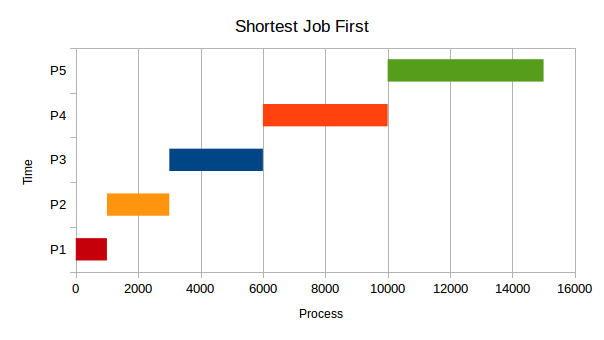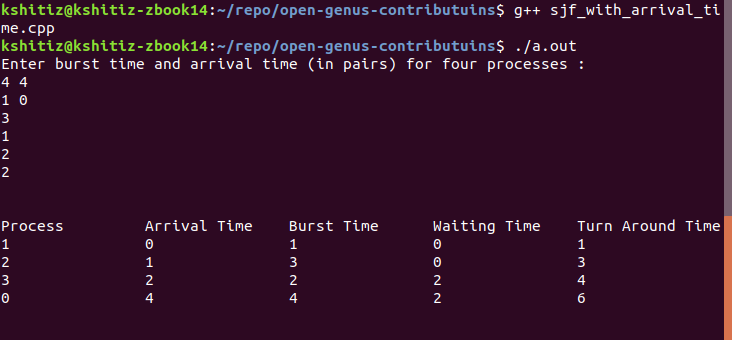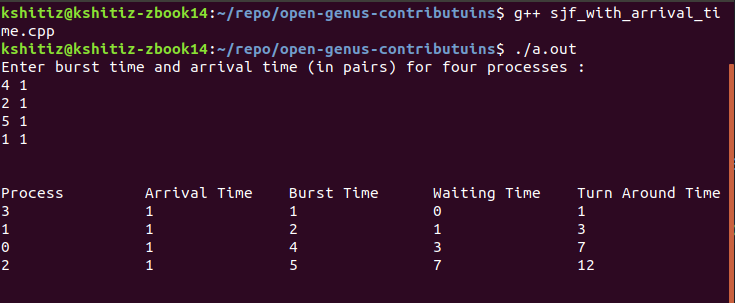×

Search anything:

# Shortest Job First CPU Scheduling algorithm

#### Software Engineering AlgorithmsGet this book -> Problems on Array: For Interviews and Competitive Programming

Reading time: 40 minutes | Coding time: 15 minutes

Shortest Job First (SJF) CPU scheduling algorithm is a CPU scheduling algorithm which is based on the principles of Greedy Algorithms. The key idea is to allocate the CPU to the process with the smallest burst time so that the CPU seems to be more responsive. Burst time is the amount of time required by a process for its execution on the CPU.

It is a Non-Preemptive CPU Scheduling algorithm. We have provided an implementation of SJF algorithm in C++ as well.

It is difficult to predict the burst time so it is difficult to implement this algorithm. In real systems, different approaches can be taken to predict burst time and some systems have been found to be using Machine Learning algorithms to predict it based on previous usage of the system.

• Maximum throughput.

Throughput means total number of processes executed per unit time. Shortest job first scheduling algorithm selects the waiting process with the smallest execution time. Thus, in SLF, shortest jobs are executed first making the CPU utilization maximum. So, maximum number of tasks are completed.

• Minimum waiting and turn around time as compared with other scheduling algorithms.

• It may cause starvation if shorter process keeps coming.

In case process with lower burst time appears before process with higher burst time then only the starvation may occur, since the algorithm will always choose the process with lowest Burst Time, the process with higher Burst Time will never be able to get the share of CPU.

• Very hard to implement as Operating System cannot calculate the burst time of every process and will not be able to sort the processes.

## Algorithm of SJF

1. Sort all the processes according to their arrival time.
2. Select the process with minimum arrival time as well as minimum burst time.
3. After completion of the process, select from the ready queue the process which has the minimum burst time.
4. Repeat above processes untill all processes have finished their execution.## Explaning procedure as per SJF algorithm

Process Arrival time Burst time Waiting time Turn around time
P1 1 7 0 7
P2 3 3 7 10
P3 6 2 2 4
P4 7 10 14 24
P5 9 8 4 12

Since arrival time of any process is not 0, there will be no execution or allocation of CPU from time 0 to 1.

Following the algorithm further, process having the least burst time among the available processes will be executed. Till now, we have only one process in the ready queue hence the process will be scheduled no matter what the burst time is.

The process will be executed till 8 units of time. Till then three more processes have arrived in the ready queue therefore, the process with the lowest burst time will be choosen. P3 will be executed next as it has lowest burst time. So that's how the process will go on in shortest job first (SJF) scheduling algorithm.

## Code Explanation

1. First we ask the user to enter the required values like : Burst Time and Arrival Time. Also, the processes are assigned with a unique process id.
``````cout<<"Enter burst time for four processes : "<<endl;
for(int i=0;i<4;i++)
{
process[i]=i;
//Process is assigned with process number
cin>>burst_time[i];
//Burst time is requested by the user
}
``````

Here, the users are asked to enter relevant details for the working of program like burst time which is essential for the working of the code.

1. Then we sort the processes according to arrival time.
``````for(int i=0;i<4;i++)
//For loop is used to iterate through all processes
{
for(int j=i+1;j<4;j++)
//Nested for loop is used to compare current process with all the further processes
{
if(arrival_time[i]>arrival_time[j])
//Arrival time of two processes are compared and checked if arrival time of current process is greater than that of further process
{
swap(process[i],process[j]);
swap(arrival_time[i],arrival_time[j]);
swap(burst_time[i],burst_time[j]);
//Process id, arrival time as well as burst time of the processes are sorted inaccordance with arrival time by swapping then accordingly.
}
}
}
``````

Here, the processes are sorted according to their arrival time in accending order.

1. After sorting according to arrival time, we sort processes according to burst time, in case, the arrival time is same.
``````for(int i=0;i<4;i++)
//For loop is used to iterate through all processes
{
for(int j=i+1;j<4;j++)
//Nested for loop is used to compare current process with all the further processes
{
if(arrival_time[i]==arrival_time[j])
//Condition is checked if two processes have same arrival time.
{
if(burst_time[i]>burst_time[j])
// In case the arrival time is same, the processes are compared according to their burst time and further sorted.
{
swap(process[i],process[j]);
swap(arrival_time[i],arrival_time[j]);
swap(burst_time[i],burst_time[j]);
//Process id, arrival time as well as burst time of the processes are sorted inaccordance with burst time if they have same arrival time by swapping then accordingly.
}
}
}
}
``````

Here, processes with same arrival time are sorted further in accordance to their burst time.

1. After the sorting process is complete, the operations are performed to calculate completion time, turn around time and waiting time.

Processes - are performed to calculate completion time turn around time and waiting time.

1. For the first process, the completion time is initialised as the sum of burst time and arrival time of the first process.
``````completion_time = arrival_time + burst_time;
turn_around_time = completion_time - arrival_time;
waiting_time = turn_around_time - burst_time;
``````

Here, the values for first process are calculated.

1. For further processes, we first check if the arrival time of next process is greater than the completion time of previous process.
``````for(int i=1;i<4;i++)
// Process from the second are iterated to till the last process in reached.
{
temp = completion_time[i-1];
int low = burst_time[i];
for(int j=i;j<4;j++)
{
if(temp >= arrival_time[j] && low >=burst_time[j])
// Completion time of previous process is compare with the arrival time of current process as well the burst time is compared.
{
low = burst_time[j];
value = j;
}
}
``````

Here the processes are compared for smaller burst time and the process with smaller burst time is executed first.

1. If so, then the completion time is the sum of arrival time of the process and the burst time.

2. Else, the completion time is the sum of completion time of previous process and the burst time of current process.

3. Further the turn around time and waiting time of processes are calculated considering turn around time as the difference of completion time and arrival time of the given process. Also, the waiting time is calculated as the difference of turn around time and butst time of the current process.

``````completion_time[value] = temp + burst_time[value];
turn_around_time[value] = completion_time[value] - arrival_time[value];
waiting_time[value] = turn_around_time[value] - burst_time[value];
// Value of completion time, waiting time and turn around time is calculated using formulae.
``````

For the executing process, we calculate the values.

1. The processes 6 to 9 are then repeated till all the processes are completed.

## Combined Program for SJF CPU Scheduling (with Arrival Time)

Following is the complete C++ code:

``````#include <iostream>
#include <stdlib.h>

using namespace std;

void input_burst_arrival_time(int burst_time[],int process[],int arrival_time[])
{
cout<<"Enter burst time and arrival time (in pairs) for four processes : "<<endl;
for(int i=0;i<4;i++)
// The user is asked to enter burst time and arrival time of 4 processes.
{
process[i]=i;
cin>>burst_time[i];
cin>>arrival_time[i];
// Input is taken for the butst time and arrival time.
}
}

void sort_arrival_time(int arrival_time[],int process[],int burst_time[])
// Function is used to sort the processes on the basis of their arrival time.
// In case, two processes have same arrival time they will be placed next to each other.
{
for(int i=0;i<4;i++)
{
for(int j=i+1;j<4;j++)
{
if(arrival_time[i]>arrival_time[j])
{
swap(process[i],process[j]);
swap(arrival_time[i],arrival_time[j]);
swap(burst_time[i],burst_time[j]);
// Process id, arrival time as well as burst time of the processes are sorted inaccordance with arrival time.
}
}
}
}

void sort_burst_time(int arrival_time[],int process[],int burst_time[])
// Function is used to sort the processes with same burst time according to their arrival time.
{
for(int i=0;i<4;i++)
{
for(int j=i+1;j<4;j++)
{
if(arrival_time[i]==arrival_time[j])
// Condition is checked if two processes have same arrival time.
{
if(burst_time[i]>burst_time[j])
// In case the arrival time is same, the processes are compared according to their burst time and further sorted.
{
swap(process[i],process[j]);
swap(arrival_time[i],arrival_time[j]);
swap(burst_time[i],burst_time[j]);
}
}
}
}
}

void sjf_operations(int arrival_time[],int waiting_time[],int burst_time[],int turn_around_time[],int completion_time[])
// This function is used to calcuate waiting time and turn around time fot the processes.
{
int temp;
int value;

completion_time = arrival_time + burst_time;
turn_around_time = completion_time - arrival_time;
waiting_time = turn_around_time - burst_time;
// For the first process the completion time, waiting time an turn around time are initialised.

for(int i=1;i<4;i++)
// Process from the seconf are iterated to till the last process in reached.
{
temp = completion_time[i-1];
int low = burst_time[i];
for(int j=i;j<4;j++)
{
if(temp >= arrival_time[j] && low >=burst_time[j])
// Completion time of previous process is compare with the arrival time of current process as well the burst time is compared.
{
low = burst_time[j];
value = j;
}
}
completion_time[value] = temp + burst_time[value];
turn_around_time[value] = completion_time[value] - arrival_time[value];
waiting_time[value] = turn_around_time[value] - burst_time[value];
// Value of completion time, waiting time and turn around time is calculated using formulae.
}
}

void print_table(int process[],int burst_time[],int waiting_time[],int turn_around_time[],int arrival_time[])
// Table is printed which prints the calculated value as the output.
{
cout<<endl<<endl;

cout<<"Process"<<" \t"<<"Arrival Time"<<" \t"<<"Burst Time"<<" \t"<<"Waiting Time"<<" \t"<<"Turn Around Time"<<endl;
for(int i=0;i<4;i++)
{
cout<<process[i]<<"\t\t"<<arrival_time[i]<<"\t\t"<<burst_time[i]<<"\t\t"<<waiting_time[i]<<"\t\t"<<turn_around_time[i]<<endl;
}

cout<<endl<<endl;
}

int main()
{
int process;
int burst_time;
int waiting_time;
int completion_time;
int turn_around_time;
int arrival_time;

input_burst_arrival_time(burst_time,process,arrival_time);

sort_arrival_time(arrival_time,process,burst_time);

sort_burst_time(arrival_time,process,burst_time);

sjf_operations(arrival_time,waiting_time,burst_time,turn_around_time,completion_time);

print_table(process,burst_time,waiting_time,turn_around_time,arrival_time);

return 0;
}
``````

After compiling and running the output is :